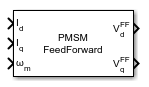Main Content

# PMSM Feed Forward Control

Decouple d-axis and q-axis current to eliminate disturbance

• Library:
• Motor Control Blockset / Controls / Control Reference

•## Description

The PMSM Feed Forward Control block decouples d-axis and q-axis current controls and generates the corresponding feed-forward voltage gains to enable field-oriented control of Permanent Magnet Synchronous Motor (PMSM).

The block accepts feedback values of d-axis and q-axis currents and the mechanical speed of the rotor.

### Equations

If you select `Per-Unit (PU)` in the Input units parameter, the block converts the inputs to SI units before performing any computation. After calculating the output, the block converts the values back to per unit values.

These equations describe the computation of feed-forward gain by the block.

`${V}_{d}^{FF}={\omega }_{\text{e}}{\lambda }_{\text{d}}={\omega }_{\text{e}}{L}_{\text{d}}{I}_{\text{d}}+{\omega }_{\text{e}}{\lambda }_{\text{m}}$`
`${V}_{q}^{FF}=-{\omega }_{\text{e}}{\lambda }_{\text{q}}=-{\omega }_{\text{e}}{L}_{\text{q}}{I}_{\text{q}}$`

For detailed set of equations and assumptions that Motor Control Blockset™ uses for a PMSM, see Mathematical Model of PMSM.

where:

• ${\omega }_{e}$ is the electrical speed corresponding to frequency of stator voltages (Radians/ sec).

• ${L}_{\text{d}}$ and ${L}_{\text{q}}$ are the d-axis and q-axis stator winding inductances (Henry).

• ${I}_{\text{d}}$ and ${I}_{\text{q}}$ are the d-axis and q-axis currents (Amperes).

• ${\lambda }_{\text{d}}$ and ${\lambda }_{\text{q}}$ are the magnetic fluxes along the d- and q-axes (Weber).

• ${\lambda }_{\text{m}}$ is the permanent magnet flux linkage (Weber).

## Ports

### Input

expand all

Current along the d-axis of the rotating dq reference frame.

Data Types: `single` | `double` | `fixed point`

Current along the q-axis of the rotating dq reference frame.

Data Types: `single` | `double` | `fixed point`

Mechanical speed of the rotor.

Data Types: `single` | `double` | `fixed point`

### Output

expand all

Feed-forward voltage gain along the d-axis of the rotating dq reference frame.

Data Types: `single` | `double` | `fixed point`

Feed-forward voltage gain along the q-axis of the rotating dq reference frame.

Data Types: `single` | `double` | `fixed point`

## Parameters

expand all

Number of pole pairs available in the motor.

Stator winding inductance (in Henry) along the direct-axis of the rotating dq reference frame.

Stator winding inductance (in Henry) along the quadrature-axis of the rotating dq reference frame.

Peak permanent magnet flux linkage (in Weber).

Saturation limit (in Volts) for the output voltages `VdFF` and `VqFF`.

Unit of the input values.

Base voltage (in Volts) for per-unit system.

#### Dependencies

To enable this parameter, set Input units to `Per-Unit (PU)`.

Base current (in Amperes) for per-unit system.

#### Dependencies

To enable this parameter, set Input units to `Per-Unit (PU)`.

Base speed (in rpm) for per-unit system.

#### Dependencies

To enable this parameter, set Input units to `Per-Unit (PU)`.

## See Also

### Topics

Introduced in R2020a

Get ebook